# NCERT Solutions for Class 9 Science Motion part 2## myCBSEguide App

CBSE, NCERT, JEE Main, NEET-UG, NDA, Exam Papers, Question Bank, NCERT Solutions, Exemplars, Revision Notes, Free Videos, MCQ Tests & more.

NCERT solutions for Science Motion## NCERT Class 9 Science Chapter wise Solutions

• 01 –  Matter in Our Surroundings
• 02 – Is Mattter Around us Pure
• 03 – Atoms and Molecules
• 04 – Structure of the Atom
• 05 – The Fundamental Unit of Life
• 06 – Tissues
• 07 – Diversity in Living Organisms
• 08 – Motion
• 09 – Force and Laws of Motion
• 10 – Gravitation
• 11 – Word and Energy
• 12 – Sound
• 13 – Why Do We Fall Ill
• 14 – Natural Resources
• 15 – Improvement in Food Resources

## NCERT Solutions for Class 9 Science Motion part 2

###### 1. An athlete completes one round of a circular track of diameter 200 m in 40 s. What will be the distance covered and the displacement at the end of 2 minutes 20 s?

Ans. circumference of circular track = 2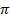r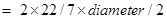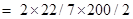= 4400/7 m

rounds completed by athlete in 2min20sec = s= 140/40 = 3.5

therefore, total distance covered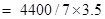= 2200 m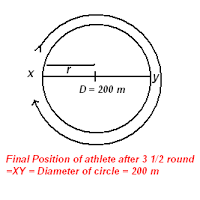Since one complete round of circular track needs 40s so he will complete 3 rounds in 2mins and in next 20s he can complete half round therefore displacement = diameter = 200m.

###### 2. Joseph jogs from one end A to the other end B of a straight 300 m road in 2 minutes 50 seconds and then turns around and jogs 100 m back to point C in another 1 minute. What are Joseph’s average speeds and velocities in jogging (a) from A to B and (b) from A to C?

Ans. (a) distance = 300m

time = 2min30seconds = 150 seconds

average speed from A to B = average velocity from A to B

= 300m/150s = 2m/s

(b) average speed from A to C = (300+100)m/(150+60)sec

= 400m/210s = 1.90m/s

displacement from A to C = (300 – 100)m =200m

time =2min30sec + 1min = 210s

velocity = displacement/time = 200m/210s = 0.95m/s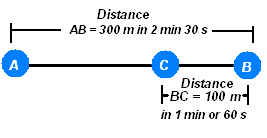NCERT Solutions for Class 9 Science Motion part 2

###### 3. Abdul, while driving to school, computes the average speed for his trip to be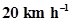. On his return trip along the same route, there is less traffic and the average speed is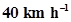. What is the average speed for Abdul’s trip?

Ans. If we suppose that distance from Abdul’s home to school = x kms

while driving to school :-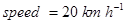,

velocity = displacement/time

20 = x/t, or, t=x/20 hr

on his return trip :-

speed = 40 km h–1 ,

40= x /t’

or, t’ =x/40 hr

total distance travelled = x + x = 2x

total time = t + t’ = x/20 + x/40 =(2x + x)/40 = 3x/40 hr

average speed for Abdul’s trip = 2x/(3x/40) = 80x/3x = 26.67km/hr

NCERT Solutions for Class 9 Science Motion part 2

###### 4. A motorboat starting from rest on a lake accelerates in a straight line at a constant rate of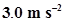for 8.0 s. How far does the boat travel during this time?

Ans. since the motorboat starts from rest so u= 0

time (t) = 8s,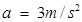distance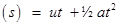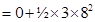= 96m

###### 5. A driver of a car travelling at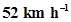applies the brakes and accelerates uniformly in the opposite direction. The car stops in 5 s. Another driver going at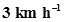in another car applies his brakes slowly and stops in 10 s. On the same graph paper, plot the speed versus time graphs for the two cars. Which of the two cars travelled farther after the brakes were applied?

Ans.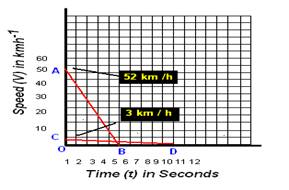As given in the figure below AB (in red line) and CD(in red line) are the Speed-time graph for given two cars with initial speeds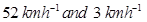respectively.

Distance Travelled by first car before coming to rest =Area of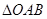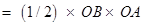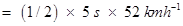=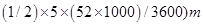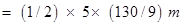= 325/9 m

= 36.11 m

Distance Travelled by second car before coming to rest =Area of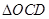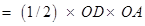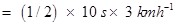=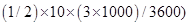m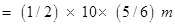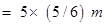= 25/6 m

= 4.16 m

∴ Clearly the first car will travel farther (36.11 m) than the first car(4.16 m).

NCERT Solutions for Class 9 Science Motion part 2

###### 6. Fig 8.11 shows the distance-time graph of three objects A, B and C. Study the graph and answer the following questions: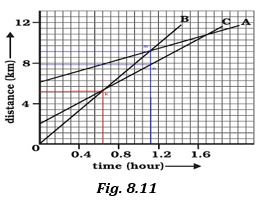(a) Which of the three is travelling the fastest?

(b) Are all three ever at the same point on the road?

(c) How far has C travelled when B passes A?

(d) How far has B travelled by the time it passes C?

Ans.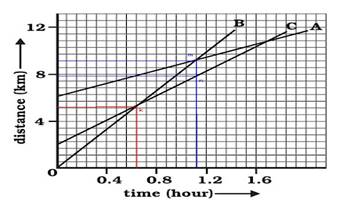(a) It is clear from graph that B covers more distance in less time. Therefore, B is the fastest.

(b) All of them never come at the same point at the same time.

(c) According to graph; each small division shows about 0.57 km.

A is passing B at point S which is in line with point P (on the distance axis) and shows about 9.14 km

Thus, at this point C travels about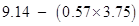km = 9.14 km – 2.1375 km = 7.0025 km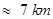Thus, when A passes B, C travels about 7 km.

(d) B passes C at point Q at the distance axis which is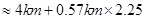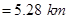Therefore, B travelled about 5.28 km when passes to C.

NCERT Solutions for Class 9 Science Motion part 2

###### 7. A ball is gently dropped from a height of 20 m. If its velocity increases uniformly at the rate of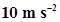, with what velocity will it strike the ground? After what time will it strike the ground?

Ans. Let us assume, the final velocity with which ball will strike the ground be ‘v’ and time it takes to strike the ground be ‘t’

Initial Velocity of ball u=0

Distance or height of fall s=20m

Downward acceleration a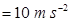As we know,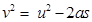Or, 2as =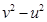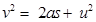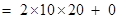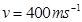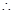Final velocity of ball, v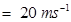t= (v-u)/aTime taken by the ball to strike= (20-0)/10

= 20/10

= 2 seconds

NCERT Solutions for Class 9 Science Motion part 2

###### 8. The speed-time graph for a car is shown is Fig. 8.12.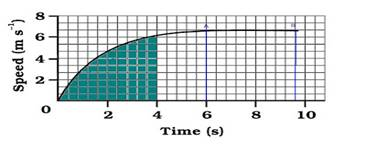Fig. 8.12

(a) Find how far does the car travel in the first 4 seconds. Shade the area on the graph that represents the distance travelled by the car during the period.

(b) Which part of the graph represents uniform motion of the car?

Ans.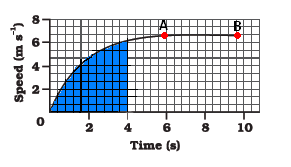(a) Distance travelled by car in the 4 second

The area under the slope of the speed – time graph gives the distance travelled by an object.

In the given graph

56 full squares and 12 half squares come under the area slope for the time of 4 second.

Total number of squares = 56 + 12/2 = 62 squares

The total area of the squares will give the distance travelled by the car in 4 second. on the time axis,

5 squares = 2seconds, therefore 1 square = 2/5 seconds

on speed axis there are 3 squares = 2m/s

therefore, area of one square =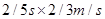= 4/15 m

so area of 62 squares=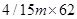= 248/15 m = 16.53 m

Hence the car travels 16.53m in the first 4 seconds.

(b) The straight line part of graph, from point A to point B represents a uniform motion of car.

NCERT Solutions for Class 9 Science Motion part 2

###### 9. State which of the following situations are possible and give an example for each of these:

(a) an object with a constant acceleration but with zero velocity

(b) an object moving in a certain direction with an acceleration in the perpendicular direction.

Ans. (a) An object with a constant acceleration can still have the zero velocity. For example an object which is at rest on the surface of earth will have zero velocity but still being acted upon by the gravitational force of earth with an acceleration of 9.81 ms-2 towards the center of earth. Hence when an object starts falling freely can have contant acceleration but with zero velocity.

(b) When an athlete moves with a velocity of constant magnitude along the circular path, the only change in his velocity is due to the change in the direction of motion. Here, the motion of the athlete moving along a circular path is, therefore, an example of an accelerated motion where acceleration is always perpendicular to direction of motion of an object at a given instance. Hence it is possible when an object moves on a circular path.

NCERT Solutions for Class 9 Science Motion part 2

###### 10. An artificial satellite is moving in a circular orbit of radius 42250 km. Calculate its speed if it takes 24 hours to revolve around the earth.

Ans. Let us assume an artificial satellite, which is moving in a circular orbit of radius 42250 km covers a distance ‘s’ as it revolve around earth with speed ‘v’ in given time ‘t’ of 24 hours.

= 42250 km

Radius of circular orbit r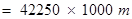Time taken by artificial satellite t=24 hours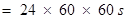Distance covered by satellite s =Circumference of circular orbit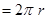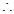Speed of satellite v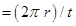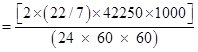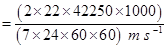]

=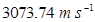= 3.073 km/s

## NCERT Solutions for Class 9 Science

NCERT Solutions Class 9 Science PDF (Download) Free from myCBSEguide app and myCBSEguide website. Ncert solution class 9 Science includes text book solutions .NCERT Solutions for CBSE Class 9 Science have total 15 chapters. 9 Science NCERT Solutions in PDF for free Download on our website. Ncert Science class 9 solutions PDF and Science ncert class 9 PDF solutions with latest modifications and as per the latest CBSE syllabus are only available in myCBSEguide.

## CBSE app for Class 9

To download NCERT Solutions for class 9 Science, Computer Science, Home Science,Hindi ,English, Maths Social Science do check myCBSEguide app or website. myCBSEguide provides sample papers with solution, test papers for chapter-wise practice, NCERT solutions, NCERT Exemplar solutions, quick revision notes for ready reference, CBSE guess papers and CBSE important question papers. Sample Paper all are made available through the best app for CBSE students and myCBSEguide website.### 1 thought on “NCERT Solutions for Class 9 Science Motion part 2”

1. The answer is right but it is a very hard method to solve the problem
This methods confused the child that what is right and what is wrong